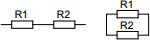# Resistor finder

## Finds a combination of two series or parallel connected resistors for a custom value. Returns a list of the best values found.

 Example 1: Must calculate the combination of 1% resistors to produce a resistance of 66 kilo-ohms View example
 Desired resistance ohms E-series E6 (20%)E12 (10%)E24 (5%)E48 (2%)E96 (1%)E192 (>1%)You may use one of the following SI prefix after a value: p=pico, n=nano, u=micro, m=milli, k=kilo, M=mega, G=giga

Fill all the values of the following
• Desired resistance - Target resistance. Can be anything in between 1 ohm and 10 megaohm.
• E-series - Resistors E-series to use